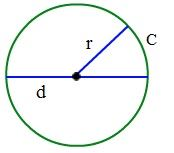# What is Circle? Formula of Circle

Circle is a particular shape and defined as the set of points in a plane placed at equal distance from a single point called the center of the circle. We use the circle formulas to calculate the area, diameter, and circumference of a circle. The length between any point on the circle and its center is known as its radius. Any line that passes through the center of the circle and connects two points of the circle is known as the Diameter of the circle. Radius is half the length of a diameter of the circle. Area of the circle describes the amount of space covered by the circle and the length of the boundary of the circle is known as its Circumference.• r denotes the radius of the circle.
• d indicates the diameter of the circle.
• c indicates circumference of the circle.

### Formulas of Circles

The Formulas are Explained below as,

 Diameter of a Circle D = 2 × r Circumference of a Circle C = 2 × π × r Area of a Circle A = π × r2

### Example Question Using the Circle Formulas

Example 1

A circle has a radius 5 cm. Finds its Diameter, Area and Circumference.

Given parameters are,

Diameter given by

2r

= 2 × 5 cm

= 10 cm

Area is given by

π r2

= π × 25

= 78.57 cm2

Circumference is given by

2 π r

= 2 × π × 5

= 31.4272 cm.

Example 2

Find the Diameter, Area and Circumference of a circle of radius 15 cm.

Given parameters are

Diameter is given by

2r

= 2 × 15

= 30 cm

Area is given by

π r2

= π ×152

= π × 225

= 706.95 cm2

Circumference

= 2 π r

= 2 × π × 15

= 94.26 cm.